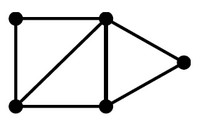# Linear Algebra - Graph

## Minimum Spanning Forest

### Formulation

Formulating GF(2) vector: subset {Pembroke, Main, Gregorian} is represented by:

Pembroke Athletic Bio-Med Main Keeney Wriston Gregorian
1 1 1
• Edge Representation in term of vectors:
Edge Vector
Pembroke Athletic Bio-Med Main Keeney Wriston Gregorian
{Pembroke, Athletic} 1 1
{Pembroke, Bio-Med} 1 1
{Athletic, Bio-Med} 1 1
{Main, Keeney} 1 1
{Main, Wriston} 1 1
{Keeney, Wriston} 1 1
{Keeney, Gregorian} 1 1
{Wriston, Gregorian} 1 1
• The vector representing {Keeney, Gregorian} is the sum, for example, of the vectors representing {Keeney, Main }, {Main, Wriston}, and {Wriston, Gregorian}.
• A vector with 1’s in entries x and y is the sum of vectors corresponding to edges that form an x-to-y path in the graph. For instance, the span of the vectors representing {Pembroke, Bio-Med}, {Main, Wriston}, {Keeney, Wriston}, {Wriston, Gregorian }
• contains the vectors corresponding to
``````{Main, Keeney}, {Keeney, Gregorian}, and {Main, Gregorian}
```
```
• but not the vectors corresponding to
````{Athletic, Bio-Med } or {Bio-Med, Main}`
```

### Algorithm

``````def Grow(G)
S := 0;
consider the edges in increasing order
for each edge e:
if e’s endpoints are not yet connected
```
``````def Grow(V)
S = 0;
repeat while possible:
find a vector v in V not in Span S,
and put it in S.```
```
• Considering edges e of G corresponds to considering vectors v in V
• Testing if e’s endpoints are not connected corresponds to testing if v is not in Span S.

The Grow algorithm for MSF is a specialization of the Grow algorithm for vectors. Same for the Shrink algorithms.

## Basis

One kind of basis in a graph G: a set S of edges forming a spanning forest.

• Spanning: for each edge xy in G, there is an x-to-y path consisting of edges of S.
• Independent: no cycle consisting of edges of S

A basis for a graph is a spanning forest. Unique Representation shows that, for each edge xy in the graph,

• there is an x-to-y path in the spanning forest, and
• there is only one such path.

Discover MoreGraph - (Edge | Links | Arcs | Lines | Arrows) - Association

An edge model a relationship betweentwo node in a graph. Every edge model therefore a binary relationship (relationship between two elements) . An Edge is also known as: Links Arcs Lines Arrows...Graph - Analysis

Analysis of graphs involves the application of algorithms determining certain details the graph structure, Most of this algorithm are implemented in linear algebra. Determining all routes ...Graph - Persistence on file (format)

How to persist a graph on disk (ie in a file) GraphML () DOT_(graph_description_language)DOT file a matrix / CSV file where each row...Linear Algebra - Linear Dependency

Vectors v1, . . . , vn are linearly dependent if the zero vector can be written as a nontrivial linear combination of the vectors: In this case, we refer to the linear combination as a linear dependency...